## 【2】激活函数与Loss函数求导_loss求导_Clichong的博客-程序员宅基地

### 文章目录

1）初始点的设置

2）学习率的设置，可能会影响收敛的精度与速度

ps : 处于一个全局极小值或者是鞍点的状态时，往往会影响实验效果

``````import torch
import torch.nn.functional as F
import matplotlib.pyplot as plt
``````
``````# Jupyter notebook 中的魔法命令
%matplotlib inline                            # 在下面的代码模块里面都可以不用写 plt.show()
%config InlineBackend.figure_format = "png"   # 设置矢量图的方式来提高图片显示质量
# 常用的设置图片分辨率的有以下几个：
# %config InlineBackend.figure_format = “png"
# %config InlineBackend.figure_format = “svg"
# %config InlineBackend.figure_format = “retina"
``````

### 1.常见的激活函数与梯度

##### Sign函数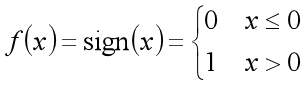``````# 使用函数的运行速度较慢
# def Single_layer(x):
#     if x <= 0:  return 0
#     else:       return 1

X = torch.linspace(-10,10,100)
Y = torch.tensor([0 if x<=0 else 1 for x in X])
plt.plot(X,Y),plt.xlabel('X_value'),plt.ylabel('Y_value'),plt.title('\$sign\$')
``````
``````([<matplotlib.lines.Line2D at 0x296e3813c88>],
Text(0.5, 0, 'X_value'),
Text(0, 0.5, 'Y_value'),
Text(0.5, 1.0, '\$sign\$'))
``````##### Sigmoid函数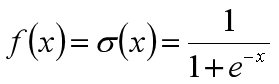``````X = torch.linspace(-3,3,100)
Y = F.sigmoid(X)
plt.plot(X,Y),plt.xlabel('X_value'),plt.ylabel('Y_value'),plt.title('\$sigmoid\$')
``````
``````([<matplotlib.lines.Line2D at 0x296e1e1d1c8>],
Text(0.5, 0, 'X_value'),
Text(0, 0.5, 'Y_value'),
Text(0.5, 1.0, '\$sigmoid\$'))
``````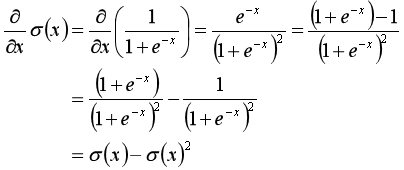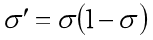##### Tanh函数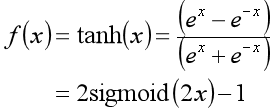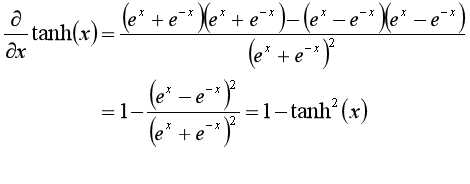``````X = torch.linspace(-3,3,100)
Y = F.tanh(X)
plt.plot(X,Y),plt.xlabel('X_value'),plt.ylabel('Y_value'),plt.title('\$tanh\$')
``````
``````([<matplotlib.lines.Line2D at 0x296e3903688>],
Text(0.5, 0, 'X_value'),
Text(0, 0.5, 'Y_value'),
Text(0.5, 1.0, '\$tanh\$'))
``````##### ReLU函数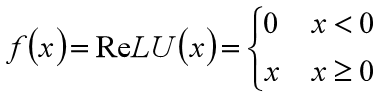``````X = torch.linspace(-3,3,100)
Y = F.relu(X)
plt.plot(X,Y),plt.xlabel('X_value'),plt.ylabel('Y_value'),plt.title('\$ReLU\$')
``````
``````([<matplotlib.lines.Line2D at 0x296e3f2e6c8>],
Text(0.5, 0, 'X_value'),
Text(0, 0.5, 'Y_value'),
Text(0.5, 1.0, '\$ReLU\$'))
``````##### Softmax函数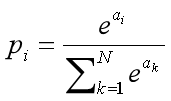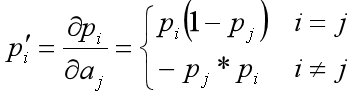##### Leaky ReLU函数

Leaky ReLU函数是在ReLU函数上面做出一个改进，用于对于ReLU函数函数来说，当x<0的时候，其梯度是为0的，而这种情况也可能会导致梯度长时间得不到更新，所以Leaky ReLU函数会在x<0的时候做一个小小的偏移，让其有一个比较小的梯度

``````X = torch.arange(-10,10,0.1)
Y = F.leaky_relu(X)
plt.xticks(range(-10,10,1))
plt.plot(X,Y),plt.xlabel('X_value'),plt.ylabel('Y_value'),plt.title('\$Leaky ReLU\$')
``````
``````([<matplotlib.lines.Line2D at 0x1c1caaff9c8>],
Text(0.5, 0, 'X_value'),
Text(0, 0.5, 'Y_value'),
Text(0.5, 1.0, '\$Leaky ReLU\$'))
``````##### SELU函数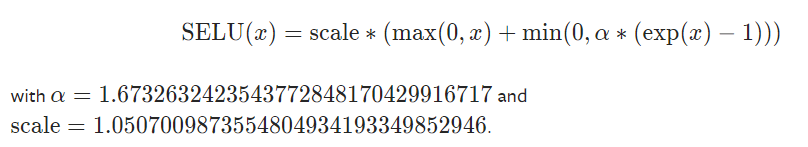``````X = torch.arange(-10,10,0.1)
Y = F.selu(X)
#cplt.xticks(range(-10,10,1))
plt.plot(X,Y),plt.xlabel('X_value'),plt.ylabel('Y_value'),plt.title('\$SeLU\$')
``````
``````([<matplotlib.lines.Line2D at 0x1c1cacac2c8>],
Text(0.5, 0, 'X_value'),
Text(0, 0.5, 'Y_value'),
Text(0.5, 1.0, '\$SeLU\$'))
``````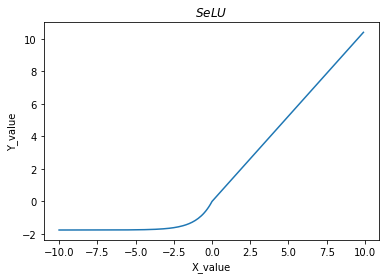##### Softplus函数

Softplus函数的表达式为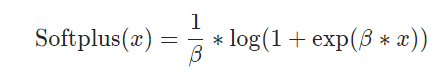``````X = torch.arange(-10,10,0.1)
Y = F.softplus(X)
plt.plot(X,Y),plt.xlabel('X_value'),plt.ylabel('Y_value'),plt.title('\$Softplus\$')
``````
``````([<matplotlib.lines.Line2D at 0x1c1cabedc48>],
Text(0.5, 0, 'X_value'),
Text(0, 0.5, 'Y_value'),
Text(0.5, 1.0, '\$Softplus\$'))
``````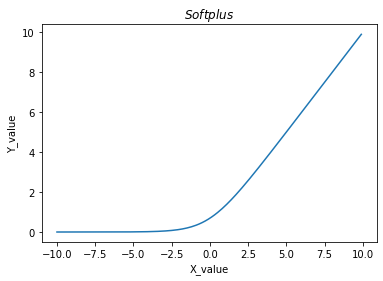### 2.常见的Loss与梯度

##### Mean Squared Error均方误差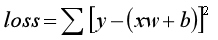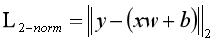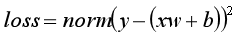``````# 代码表示为
torch.norm(y-pred,2).pow(2)
``````
``````# 现在使用torch.autograd.grad实现求导的过程
# 现在假设需要解决的任务方程是简单的一元函数：y = xw + b
# 所以其loss function为 loss = ∑[(xw + b) - y]**2

# 第一个例子：
x = torch.tensor(5)     # 设置x为5
w = torch.full(,10.)  # 设置斜率w为10
b = torch.tensor(2)     # 设置bios为2
y = torch.rand(1)+50    # 设置真实值为50附近的随机数，输出为50.6296
loss = F.mse_loss(x*w+b,y)
# 输出结果为：  tensor([1.8779]))
# 现在进行验证一下：（5*10+2-50.6296）**2=1.8779
# 对此时的w进行求导，但是出出现一个报错
# RuntimeError: element 0 of tensors does not require grad and does not have a grad_fn
# 表示w此时是不需要求导，错误原因是一开始的w没有设置好导数信息，所以pytorch自动标注了w不需要求导
# RuntimeError: only Tensors of floating point dtype can require gradients
# 又出现了一个错误，表示w需要是浮点数值，现在重新将w修改为w = torch.full(,10.)
# 此时想再来求导，但是还是出现了错误
# RuntimeError: element 0 of tensors does not require grad and does not have a grad_fn
# 出现错误的原因是：前一步已经更新了w，但pytorch的这个动态图还是没有更新，所以需要重新更新一个图
loss = F.mse_loss(x*w+b,y)
# 此时输出下数值：w,y,loss
#  tensor([50.5761]),
# 完成这个过程之后，就可以对w进行求导了
# 输出为(tensor([13.7037]),)
# 验证一下
# 2*5*(5*10+2-50.6296)=13.7037 与输出结果一致

# 补充：
# 以上过程进行中出现的问题，也就是w不能丘梯度的问题，其实可以从开始初始化的时候直接进行解决
# 以上的两个写法是等价的

# 第二个例子：
# 同时可以对多个未知量进行求导
x = torch.tensor(5)     # 设置x为5
y = torch.rand(1)+50    # 设置真实值为50附近的随机数，输出为50.6296
loss = F.mse_loss(x*w+b,y)
print(y,x*w+b-y,loss)
dw,db
# 输出为：((tensor([13.6066]),), (tensor(2.7213),))  验证结果是正确的
``````
``````(tensor([13.7037]),)
``````
###### 2）loss.backward的使用
``````# 还是以这个例子为例，其实backward函数会自动的对所有的参数进行求导，但是不会输出结果而是将结果保存在每一个未知量x.grad中
x = torch.tensor(5)     # 设置x为5
y = torch.rand(1)+50    # 设置真实值为50附近的随机数，输出为50.6296
loss = F.mse_loss(x*w+b,y)
print("loss:",loss,"[(x*w+b)-y]**2:",((x*w+b)-y)**2)
# 使用backward自动求导函数
loss.backward()
# 输出为：(tensor([17.7694]), tensor(3.5539))
# 验证一下
loss = F.mse_loss(x*w+b,y)
dw,db
# ((tensor([17.7694]),), (tensor(3.5539),))
# 可见，两种方法的输出结果一样
``````
``````loss: tensor(3.1575, grad_fn=<MseLossBackward>) [(x*w+b)-y]**2: tensor([3.1575], grad_fn=<PowBackward0>)
``````
###### 3）Softmax的使用
``````a = torch.rand(3,requires_grad=True)
p = F.softmax(a,dim=0)
# 预测值：
# (-0.13083266, 0.24673958999999998, -0.11590693)
# 输出为：(tensor([-0.1308,  0.2467, -0.1159]),)，符合预测值
``````
``````tensor([0.2001, 0.6048, 0.0787], requires_grad=True)
``````
##### Cross Entropy Loss交叉熵损失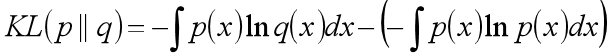• 相对熵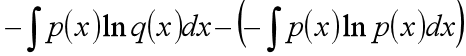• 交叉熵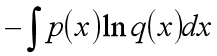若p(x) 是数据的真实概率分布，q(x)是由数据计算得到的概率分布。机器学习的目的就是希望p(x)尽可能地逼近甚至等于p(x)，从而使得相对熵接近最小值0. 由于真实的概率分布是固定的，相对熵公式的后半部分就成了一个常数。其后半部分为：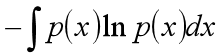那么相对熵达到最小值的时候，也意味着交叉熵达到了最小值。对q(x)的优化就等效于求交叉熵的最小值。另外，对交叉熵求最小值，也等效于求最大似然估计（maximum likelihood estimation）。

###### 1）cross_entropy的使用
``````x = torch.randn(1,28*28)
w = torch.randn(10,28*28)
# 矩阵相乘
logit = x@w.t()
# logit.shape为：torch.Size([1, 10])
# 直接求出交叉熵
F.cross_entropy(logit,torch.tensor())
# 输出为：tensor(17.2760)
``````
###### 2）nll_loss的使用

nll_loss函数其实就是最大似然函数

``````x = torch.randn(1,28*28)
w = torch.randn(10,28*28)
# 矩阵相乘
logit = x@w.t()
# softmax 处理，即将 input 转换成概率分布的形式
pred = F.softmax(logit , dim=1)
# softmax 处理处理后取对数
pred = torch.log(pred)
# 最大似然计算，其中pred是预测值，torch.tensor()是真是值也就是label值，这位置是不能换的
F.nll_loss(pred,torch.tensor())
``````

``````CrossEntropyLoss = log_sofrmax + NLLLoss
``````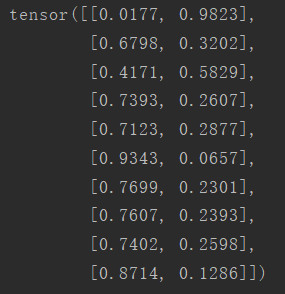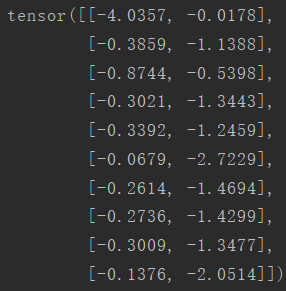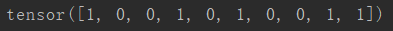``````loss = -(-0.0178 + -0.3859 + -0.8744 + /
-1.3443 + -0.3392 + -2.7229 + -0.2614 + /
-0.2736 + -1.3477 + -2.0514) / 10 = 0.96186
``````

``````print(torch.nn.CrossEntropyLosss(output, label)))
``````

### etlpy: 并行爬虫和数据清洗工具（开源）-程序员宅基地

etlpy是python编写的网页数据抓取和清洗工具，核心文件etl.py不超过500行，具备如下特点爬虫和清洗逻辑基于xml定义，不需手工编写基于python生成器，流式处理，对内存无要求内置线程池，支持串行和并行处理内置正则解析，html转义，json转换等数据清洗功能，直接输出可用文件插件式设计，能够非常方便地增加其他文件和数据库格式..._etl爬虫

### 海思编译链编译出现__aeabi_unwind_cpp_pr1重定义怎么回事-程序员宅基地

1.用arm-hisiv100nptl-linux-gcc编译代码，结果发现报错，__aeabi_unwind_cpp_pr1重定义，在librt.a先定义，使用的海思芯片是hi3520d。 2.本来以为是编译...

### BZOJ 1008--[HNOI2008]越狱（容斥&快速幂）-程序员宅基地

1008: [HNOI2008]越狱Time Limit:1 SecMemory Limit:162 MBSubmit:12593Solved:5439[Submit][Status][Discuss]Description　　监狱有连续编号为1...N的N个房间，每个房间关押一个犯人，有M种宗教，每个犯人可能信仰其中一种。如果相邻房间的犯人的宗教相同，就可能发...

### struts2 ognl 标签输出-程序员宅基地

public class TestAction extends BaseAction { private String testAttr; private Map attrMap; public Map getAttrMap() { return attrMap; } public void setAttrMap(Map attrMap) { this....